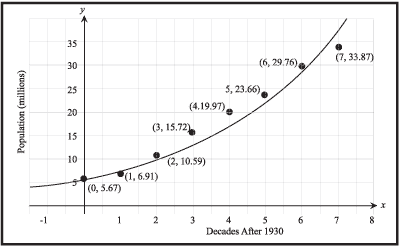# UCSMP Algebra

Main goal: The goal of UCSMP Algebra is to act as an introduction to the language of algebra, and the ways it is used in the real world, while integrating geometry, probability, and statistics with a variety of approaches and uses of contemporary technology.

Main theme I: UCSMP Algebra introduces skills, properties, uses, and representations of algebraic concepts and skills. Taking into consideration that no student learns the same way, multiple approaches to expressions, equations, and functions are presented. Topics are presented tabularly, graphically, and symbolically. Graphing calculators are used throughout and numerous CAS activities help students to develop algebraic concepts and algebraic reasoning skills. Linear, exponential, and quadratic functions are studied in detail. Powers, roots, and polynomials are included. Students are also taught how to visualize algebraic concepts physically and geometrically.Modeling the population of California with an exponential function

Main theme II: Why study algebra? Almost all concepts are developed through applications. The text explicitly discusses reasons why algebra is so important: to describe, to explain, and to prove. Algebraic expressions are used to describe patterns and explain properties of numbers, data, and geometric figures. Simple statistics are described algebraically and equations are shown to model data. Functions are presented as a way to describe change. The book culminates with a chapter in which students use algebra to prove number properties.

Main theme III: Geometry is used to assist and complement the learning of algebra. Geometric ideas such as complementary and supplementary angles, the Pythagorean Theorem, and formulas for perimeter, area, and volume are used as contexts for equation-solving and functions. Area models and other pictures are used to represent the Distributive Property and its extensions to the multiplication of polynomials. Fact triangles are employed to help students see relations between operations. Activities involve dynamic geometry systems.

Comparisons between this and earlier editions: This edition assumes that students have had experience with one-step equation solving and it moves more quickly to the study of linear equations and inequalities. Functions and function notation, found at the end of the course in previous editions, are introduced formally in the middle of the course. Graphing and CAS technology are employed throughout. Matrices are introduced in the solving of systems.

Some distinctive lessons: Explaining number puzzles (2-3); Equivalent expressions with technology (2-6);Comparing linear increase and exponential growth (7-7); Why quadratics are important (9-7); The Chi-square statistic (11-8); Solving equations as proofs (13-3).

CCSS Lessons

### Contact

UCSMP
1427 East 60th Street
Chicago, IL 60637
T: 773-702-1130
F: 773-834-4665
ucsmp@uchicago.edu